# calculate function in python jupyter notebook

1561
9
04-09-2020 03:58 PM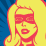Occasional Contributor

Hi everyone,

I am trying to create a script to run every night to calculate the total insects counted each day by summing two insect  count fields in a feature layer.  I can't use Arcade because the layer has "track changes" and "sync" enabled for the field crew (grrrr...). Below is my simple script to calculate the "TotalArmyworms" field based on summing the Western Yellow Armyworm field with the Bertha Armyworm field. I have tried many different ways to identify the layer from AGOL, and each time the error states that 'Item' object has no attribute 'calculate'.  Any thoughts?

``````import arcgis
import arcpy
from arcgis.gis import GIS
from arcgis import features
from arcgis.features import FeatureLayer

gis = GIS('https://arcgis.com', 'xxxxxx', 'xxxxxx')
arcpy.env.overwriteOutput = True

trap_count = gis.content.get("b6b9c68fa42b408a88c68aa9e18593d5")
trap_count

trap_count.calculate(where="fid > 0", calc_expression=[{"field": "TotalArmyworms", "sqlExpression" : "Nu_W_YellowStripedArmyworm + Nu_BerthArmyworm" }])

---------------------------------------------------------------------------
KeyError                                  Traceback (most recent call last)
C:\Program Files\ArcGIS\Pro\bin\Python\envs\arcgispro-py3\lib\site-packages\arcgis\gis\__init__.py in __getattr__(self, name)
6998         try:
-> 6999             return dict.__getitem__(self, name)
7000         except:

KeyError: 'calculate'

During handling of the above exception, another exception occurred:

AttributeError                            Traceback (most recent call last)
<ipython-input-50-2608a815f7ee> in <module>
----> 1 trap_count.calculate(where="fid > 0", calc_expression=[{"field": "TotalArmyworms", "sqlExpression" : "Nu_W_YellowStripedArmyworm + Nu_BerthArmyworm" }])
2

C:\Program Files\ArcGIS\Pro\bin\Python\envs\arcgispro-py3\lib\site-packages\arcgis\gis\__init__.py in __getattr__(self, name)
6999             return dict.__getitem__(self, name)
7000         except:
-> 7001             raise AttributeError("'%s' object has no attribute '%s'" % (type(self).__name__, name))
7002
7003     def __getitem__(self, k): # support item attributes as dictionary keys on this object

AttributeError: 'Item' object has no attribute 'calculate'

1
‍‍‍‍‍‍‍‍‍‍‍‍‍‍‍‍‍‍‍‍‍‍‍‍‍‍‍‍‍‍‍‍‍‍‍‍‍‍‍‍‍‍‍‍‍‍‍‍‍‍‍‍‍‍‍‍‍‍‍‍‍‍‍‍‍‍‍‍‍‍‍‍‍‍‍‍‍‍‍‍‍‍‍‍``````
Tags (1)
1 Solution

Accepted Solutions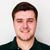byEsri Contributor

Hi Natalie,

I think you need to run calculate() on a feature layer, not the feature service. Try the code below:

``````import arcgis
import arcpy
from arcgis.gis import GIS
from arcgis import features
from arcgis.features import FeatureLayer

gis = GIS('https://arcgis.com', 'xxxxxx', 'xxxxxx')
arcpy.env.overwriteOutput = True

trap_count = gis.content.get("b6b9c68fa42b408a88c68aa9e18593d5")
trap_count

trap_count_lyr = trap_count.layers # replace the 0 with the actual layer index
````trap_count_lyr``.calculate(where="fid > 0", calc_expression=[{"field": "TotalArmyworms", "sqlExpression" : "Nu_W_YellowStripedArmyworm + Nu_BerthArmyworm" }])````

-Josh

9 RepliesbyEsri Contributor

Hi Natalie,

I think you need to run calculate() on a feature layer, not the feature service. Try the code below:

``````import arcgis
import arcpy
from arcgis.gis import GIS
from arcgis import features
from arcgis.features import FeatureLayer

gis = GIS('https://arcgis.com', 'xxxxxx', 'xxxxxx')
arcpy.env.overwriteOutput = True

trap_count = gis.content.get("b6b9c68fa42b408a88c68aa9e18593d5")
trap_count

trap_count_lyr = trap_count.layers # replace the 0 with the actual layer index
````trap_count_lyr``.calculate(where="fid > 0", calc_expression=[{"field": "TotalArmyworms", "sqlExpression" : "Nu_W_YellowStripedArmyworm + Nu_BerthArmyworm" }])````

-JoshOccasional Contributor

Thanks Josh for the answer.  I tried something similar with specifying the layer (trap_count.layers) from using a search to find the service layer (gis.content.search) and it didnt work. Im glad it was an easy fix!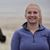Occasional Contributor III

Hi Josh,

I am hoping you might be able to help me out...I am trying to re-purpose the code above to calculate a field in a feature layer that has sync enabled. I am trying to do this in a Notebook in ArcGIS Online. Here is what my code looks like..

``````from arcgis.gis import GIS
from arcgis import features
from arcgis.features import FeatureLayer
gis = GIS("home")

gis = GIS('https://arcgis.com', 'xxxxxx', 'xxxxxx')

Site_Inspections

Site_Inspections_lyr = Site_Inspections.layers

Site_Inspections_lyr.calculate(where="OBJECTID > 0", calc_expression=[{"field": "Total_Gull_Count", "sqlExpression" : "RB_numb + HG_numb + BB_numb + Unknown_Number" }])‍‍‍‍‍‍‍‍‍‍‍‍‍‍‍‍‍‍‍‍‍‍‍‍‍‍‍‍‍‍‍‍‍‍‍‍‍‍‍``````

I keep getting the following error:

``````---------------------------------------------------------------------------
IndexError                                Traceback (most recent call last)
<ipython-input-12-b67e0c1245fc> in <module>
----> 1 Total_Gull_Count_lyr = Total_Gull_Count.layers
2
3 Total_Gull_Count_lyr.calculate(where="OBJECTID > 0", calc_expression=[{"field": "Total_Gull_Count", "sqlExpression" : "RB_numb + HG_numb + BB_numb + Unknown_Number" }])

IndexError: list index out of range

‍‍‍‍‍‍‍‍‍‍‍‍‍‍‍‍‍‍‍‍‍‍‍‍‍‍‍‍‍``````

I've triple checked my feature service, and the layer I am attempting to do this calculate on is index 1. Does it matter that this is a table?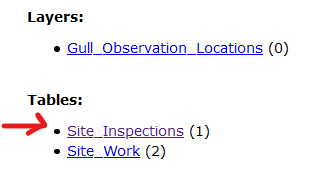Do you have any suggestions that could help out here? My goal is to schedule this to run daily to update the "Total_Gull_Count" field within my table.

Thank you,

Erica

Erica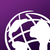New Contributor

Hi @GeoJosh

I need to calculate a field (date) based on other fields (dates) using notebook to automate this.

I am currently doing this manually once a month using

``````Case
When TA_is_Live is not null then TA_is_Live
When Data_Live_and_Onboarding is not null then Data_Live_and_Onboarding
When Signed_DSA_Returned is not null then Signed_DSA_Returned
When Discussions_Demos_Underway is not null then Discussions_Demos_Underway
When TA_Contacted is not null then TA_Contacted
Else TA_Not_Interested
End ``````

Ideally I want the notebook to do this for me. Any assistance would be great.

Many thanks

Andrew (NZ)by
Occasional Contributor

Why cant you leverage the spatial  enabled data frame? What do you want to do with the data. Visualize or republish?

``````import pandas as pd
from arcgis.gis import GIS
from arcgis import features
from arcgis.features import FeatureLayer

gis = GIS('https://arcgis.com', 'xxxxxx', 'xxxxxx')

l=item.layers
df=l.query().sdf
print(df)

#df will be output as a table

#Say you need;Total_Gull_Count
df['Total_Gull_Count']= df['RB_numb']+df['HG_numb']+ df['BB_numb'] + df['Unknown_Number']
‍‍‍‍‍‍‍‍‍‍
That will add new column. Can both be visualized or republished on portal.‍‍‍‍‍‍‍‍‍‍‍‍‍‍‍‍‍‍‍‍‍‍‍‍‍‍‍‍‍‍‍‍‍‍‍‍‍‍‍‍‍‍‍‍‍‍‍‍‍‍‍‍‍‍‍‍‍‍``````Occasional Contributor III

Hi William,

I am very new to this, so I need to look into the SEDF. My goal is to just calculate the "Total Gull Count" daily. The field already exists in the Feature Layer. The layer is edited daily in Collector and I need to tally the counts of different gull species into one total value. The "Total Gull Count" field will be used in maps and Dashboard charts/indicators. I don't want to have to re-publish the data each time; I'd like to leave it alone where it is in ArcGIS Online.

For the code sample you provided above, this still does not work when I change the layer index to 1 (this is where the "Total Gull Count" field lives - all of the values being added together also live here). I get the error "index is out of range". Does this not work with a hosted feature layer's related tables?

Thank you,

Erica

Ericaby
Occasional Contributor

For what you want to use the information I see no reason why this should not work. Output total can be input into the reports and even dashboard source files.

I cant quite resolve you error  unless I see it. Are you able to print it?Occasional Contributor

Erica - would it be easier to create a new feature layer that just represents gull counts? That would ensure that when you call :

``Site_Inspections_lyr = Site_Inspections.layers``

it would pull from the correct table.Occasional Contributor III

Hi Natalie & William,

I was able to accomplish my goal using the following:

``````import arcpy
import pandas as pd
from arcgis import features
from arcgis.features import FeatureLayerCollection
from arcgis.features import FeatureLayer
from arcgis.gis import GIS

# #### Run to process data:

gis = GIS('https://arcgis.com/', 'xxxxx', 'xxxxx')

# connect to feature layer and select proper table
item=gis.content.get('a550ded747db465eb2ff62153b559095')
l=item.tables

# need to deal with null Unknown_Number because Total_Gull_Count does not properly calculate if left null.
unknown=l.query(where="Unknown_Number IS NULL").sdf

# calculate null Unknown_Numbers to be equal to 0.
print(l.calculate(where="Unknown_Number IS NULL",
calc_expression={"field": "Unknown_Number", "sqlExpression" : '0'}))

# calculate field Total Gull Count
# select records where Total_Gull_Count is null, and then calculate value
print(l.calculate(where="Total_Gull_Count IS NULL",
calc_expression={"field": "Total_Gull_Count", "sqlExpression" : "RB_numb + HG_numb + BB_numb + Unknown_Number" }))‍‍‍‍‍‍‍‍‍‍‍‍‍‍‍‍‍‍‍‍‍‍‍‍‍‍‍‍‍‍‍‍‍‍‍‍‍‍‍‍‍‍‍‍‍‍‍‍‍‍‍‍‍‍‍‍‍‍‍‍‍‍‍‍‍‍‍‍‍‍‍‍‍‍‍‍‍‍‍``````
Erica